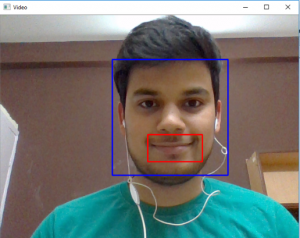# Python | Smile detection using OpenCV

Emotion detectors are used in many industries, one being the media industry where it is important for the companies to determine the public reaction to their products. In this article, we are going to build a smile detector using OpenCV which takes in live feed from webcam. The smile/happiness detector that we are going to implement would be a raw one, there exist many better ways to implement it.

Step # 1: First of all, we need to import the OpenCV library.

`import cv2`

Step #2: Include the desired haar-cascades.

Haar-cascades are classifiers that are used to detect features (of face in this case) by superimposing predefined patterns over face segments and are used as XML files. In our model, we shall use face, eye and smile haar-cascades, which after downloading need to be placed in the working directory.

All the required Haar-cascades can be found here.

 `face_cascade ``=` `cv2.CascadeClassifier(``'haarcascade_frontalface_default.xml'``) ` `eye_cascade ``=` `cv2.CascadeClassifier(``'haarcascade_eye.xml'``) ` `smile_cascade ``=` `cv2.CascadeClassifier(``'haarcascade_smile.xml'``) `

Step #3:
In this step, we are going to build main function which would be performing the smile detection.

1. The live feed coming from the webcam/video device is processed frame by frame. We process the gray scale image, as haar-cascades work better on them.
2. To detect the face, we use:

 `faces  ``=` `face_cascade.detectMultiScale(gray, ``1.3``, ``5``) `

where 1.3 is the scaling factor, and 5 is the number of nearest neighbors. We can adjust these factors as per our convenience/results to improve our detector.

3. Now for each subsequent face detected, we need to check for smiles.

 `def` `detect(gray, frame): ` `    ``faces ``=` `face_cascade.detectMultiScale(gray, ``1.3``, ``5``) ` `    ``for` `(x, y, w, h) ``in` `faces: ` `        ``cv2.rectangle(frame, (x, y), ((x ``+` `w), (y ``+` `h)), (``255``, ``0``, ``0``), ``2``) ` `        ``roi_gray ``=` `gray[y:y ``+` `h, x:x ``+` `w] ` `        ``roi_color ``=` `frame[y:y ``+` `h, x:x ``+` `w] ` `        ``smiles ``=` `smile_cascade.detectMultiScale(roi_gray, ``1.8``, ``20``) ` ` `  `        ``for` `(sx, sy, sw, sh) ``in` `smiles: ` `            ``cv2.rectangle(roi_color, (sx, sy), ((sx ``+` `sw), (sy ``+` `sh)), (``0``, ``0``, ``255``), ``2``) ` `    ``return` `frame `

Explanations –

• The face data is stored as tuples of coordinates. Here, x and y define the coordinate of the upper-left corner of the face frame, w and h define the width and height of the frame.
• The `cv2.rectangle` function takes in the arguments frame, upper-left coordinates of the face, lower right coordinates, the RGB code for the rectangle (that would contain within it the detected face) and the thickness of the rectangle.
• The `roi_gray `defines the region of interest of the face and `roi_color `does the same for the original frame.
• In line 7, we apply smile detection using the cascade.

Step #4:
We define `main `function in this step. After execution, the function can be terminated by pressing the “q” key.

 `video_capture ``=` `cv2.VideoCapture(``0``) ` `while` `True``: ` `   ``# Captures video_capture frame by frame ` `    ``_, frame ``=` `video_capture.read()  ` ` `  `    ``# To capture image in monochrome                     ` `    ``gray ``=` `cv2.cvtColor(frame, cv2.COLOR_BGR2GRAY)   ` `     `  `    ``# calls the detect() function     ` `    ``canvas ``=` `detect(gray, frame)    ` ` `  `    ``# Displays the result on camera feed                      ` `    ``cv2.imshow(``'Video'``, canvas)  ` ` `  `    ``# The control breaks once q key is pressed                         ` `    ``if` `cv2.waitKey(``1``) & ``0xff` `=``=` `ord``(``'q'``):                ` `        ``break` ` `  `# Release the capture once all the processing is done. ` `video_capture.release()                                  ` `cv2.destroyAllWindows() `

Output:My Personal Notes arrow_drop_upCheck out this Author's contributed articles.

If you like GeeksforGeeks and would like to contribute, you can also write an article using contribute.geeksforgeeks.org or mail your article to contribute@geeksforgeeks.org. See your article appearing on the GeeksforGeeks main page and help other Geeks.

Please Improve this article if you find anything incorrect by clicking on the "Improve Article" button below.

Article Tags :

4

Please write to us at contribute@geeksforgeeks.org to report any issue with the above content.